1.0  Creating a kind of artificial gravity

Just an idea

Should it be possible to create a sort of artificial gravitity/force? (for flying cars, skateboards, helicopters without roters, propelling spacecrafts ..)

Let´s consider a  rod  turning with a constant angular velocity, and a mass connected to this rod, that can be moved alongside the rod.

In position 1 the mass is at its greatest distance r1 from the axis of the rod.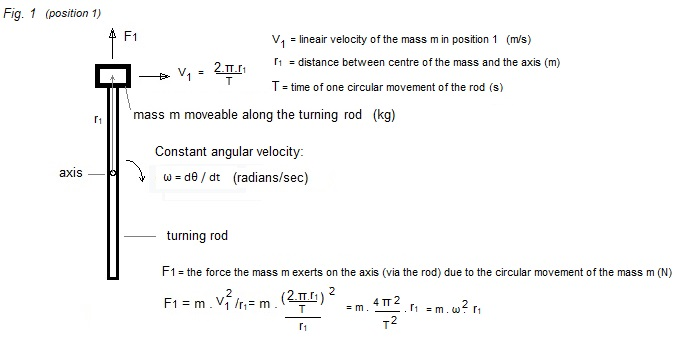For example: m = 0,5 kg;  r1 = 0,20 m;  T =  0,1 s  (10 revolutions/sec)    -> F1394,8 N

Now the rod has turned through θ = 180 °  and the mass has been moved towards the centre.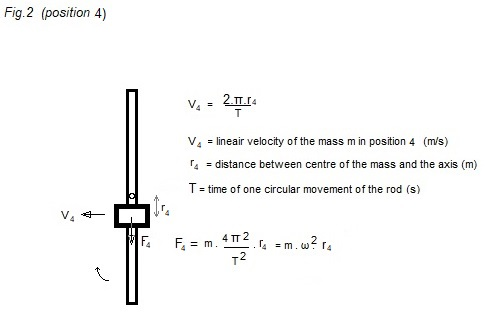For example: m = 0,5 kg;  r2 = 0,05 m;  T =  0,1 s  (10 revolutions/sec)    -> F2 98,7 N

F1 - F2  = 296,1 N (= about 30 kgf)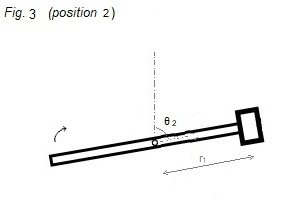The rod has turned through an angle  θ2 from position 1 to position 2 with the mass m still at its greatest distance r1 from the axis.

A mechanism connected at the rod (for example a kind of electromagnet) starts pulling the mass m towards the centre.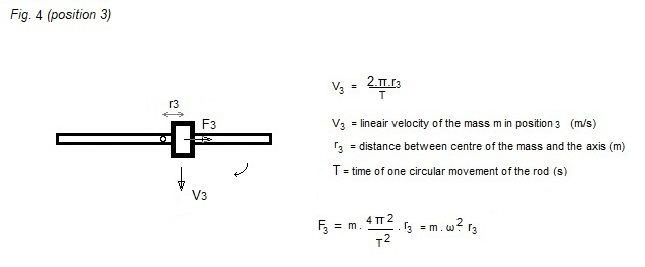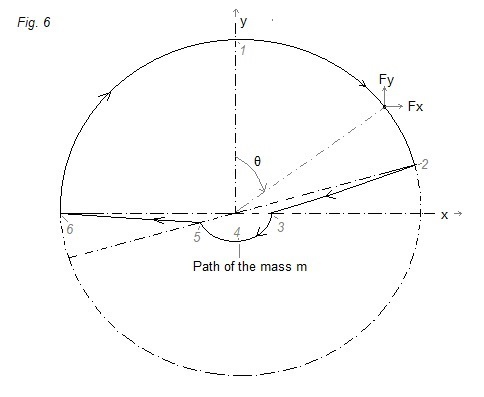In figure 6 a possible path of mass m is indicated (perhaps some points will be in reality a little bit more smoothed, but I intuit that this is not so important).

If we express the path of the mass m as a function of time, we should be able to calculate the force (as a function of time) that has to be exerted on the mass m to oblige it to follow this path.

This force will be equal, but opposite in sign to the force that the mass m exerts on the rod (on the axis of the rod)

.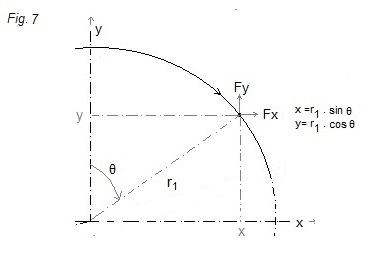θ(t) = 360/(2π) . ω.t  (in degrees; ω = 2π/T ; T = time of one revolution; the angular velocity is constant)

Programming in Pascal/Delphi:

We´ll get a net accelarion upwards?

Let´s run the program and find out ... ??????

The result are strange values..   probably cause: when the mass starts to move towards the centre, the speed alongside the radius has suddenly a value, which in reality is not possible. It should feel an accelaration towards the centre and increase the speed towards the centre as a function of time, and later on get a deaccelaration and then slowly approaching the centre.

----------------

Let´s calculate the following:  (should be quite easy to contruct..)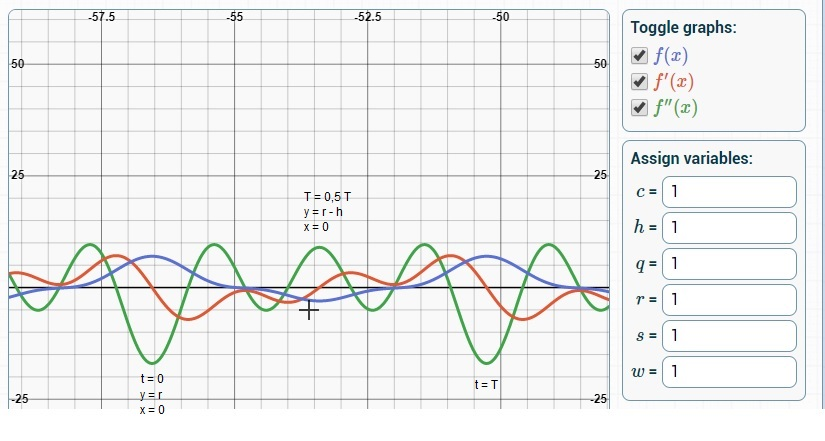The mean accelaration in the y-direction is the integral of f"(t) dt over T = > the surface of ff" under the horizontal axis = the surface of ff" above the horizontal axis.

f´(t) is the primitive function of f"t), if you follow the red curve of f´(t) you will see easily that the surface under = surface above over one period T.

=> the mean accelaration in the y-direction = zero

Newton's first law holds ...https://patents.google.com/patent/US3683707A/en  (a device to convert a circular movement into a linear force)14 February 2020      by  Rinze Joustra        www.valgetal.com